# Improve Pulse Oximetry Measurements Using Dark Current Compensation

By Bonnie Baker

Contributed By De Noord-Amerikaanse redacteurs van Digi-Key

The wearable pulse oximeter is a noninvasive medical device that measures blood oxygen saturation and pulse rate. The techniques rely upon the transmission and detection of LED light through a translucent part of the body, typically the finger.

While the technique is well understood, an accurate reading can be difficult to achieve as it depends upon many variables. On the detection side, these variables include the signal conditioning elements necessary to achieve optimum sensitivity, dynamic range and bandwidth, as well as dealing with dark current from the PIN diode. There are also problems of cost and power consumption.

As a result, it can be challenging to get the photosensor to detect high and low signals accurately.

For many designers, the best path forward is to avoid reinventing the wheel and use existing circuits. The use of pre-existing designs lowers overall cost while ensuring a maximum chance of a successful design.

This article discusses the requirements of the detection signal conditioning chain for a wearable pulse oximeter. It introduces the critical elements of that chain, including the use of a dark current compensation diode. It then describes how to get a design off the ground using a reference design that combines well-matched elements in a practical configuration.

## The pulse oximeter’s operation

A pulse oximeter continuously measures the percentage of a patient’s hemoglobin (Hgb), hemoglobin saturated with oxygen (HbO2), and pulse rate. During this measurement, a photodiode detects alternating infrared LED and red LED transmitted light through the patient’s finger, toe, or earlobe. In the patient’s blood, oxygenated hemoglobin (HbO2) absorbs the infrared LED light (940 nm), and oxygen-free hemoglobin (Hgb) absorbs the red LED light (650 nm). In a pulse oximeter, two LEDs are rapidly and sequentially excited by two current sources. A photodiode quickly detects the intensity of the light from each LED. This measurement renders a ratio between HbO2 and Hgb to create an estimate of the blood oxygen content as a percentage. The pulse rate measurement requires several samples of the pulsing blood’s waveform. To accurately measure these parameters, the high-speed, photodiode’s signal path must have both low noise and low distortion devices.

## Traditional photo sensing circuit

The standard approach to design a precision photo sensing circuit is to place a photodiode (D1) across an op amp’s CMOS or FET transistor inputs, and a resistor in parallel with a capacitor in the feedback loop. The circuit was modeled using Analog Devices’ Photo Circuit Design Wizard (Figure 1). To capture the red and infrared light an OSRAM Opto SemiconductorsSFH 2701 photodiode is used, which has an optical range of 400 nanometers (nm) to 1050 nm.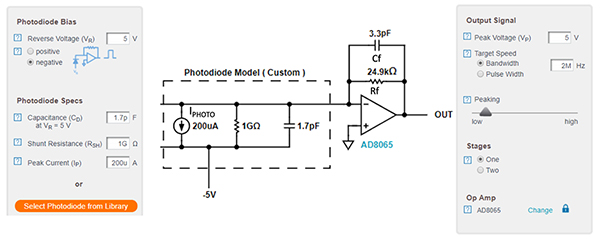Figure 1: The traditional photo sensing circuit places the photodiode (D1) across an op amp’s CMOS or FET transistor inputs, and a resistor in parallel with a capacitor in the feedback loop. (Image source: Bonnie Baker)

In Figure 1, the incident light on the photodiode causes current (IPHOTO) to flow from the diode’s cathode to the anode with a maximum value of 200 microamperes (mA). Since the input impedance of the inverting CMOS amplifier is extremely high, the photodiode captures incident light from the infrared and red LED, causing current to flow through the feedback resistor, Rf. The voltage at the inverting input of the amplifier remains at a ground potential by tracking the virtual voltage of the amplifier’s non-inverting input. Consequently, the output voltage changes in accordance with IPHOTO x Rf.

When light impinges on the photodiode, the circuit converts IPHOTO to an output voltage, per the transfer function shown in in Equation 1.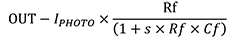Equation 1

Where:

OUT = the op amp’s output voltage

IPHOTO = photodiode current in amperes

Rf = feedback resistor in ohms

s = a complex frequency variable (jω) where ω (radians) = 2πf

Cf = feedback capacitance in Farads

It is useful to note from Equation 1 that the signal frequency pole (the frequency at which the gain decreases with increasing frequency) is equal to 2 x p x Rf x Cf.

This simple solution is often destined for failure if subtleties, such as the amplifier and photodiode parasitic capacitances, are not taken into account. For instance, a system step response could produce an output that has an unacceptable amount of ringing. Alternatively, the circuit could oscillate. If an instability problem is addressed and solved, the output response might still be too noisy for reliable results.

Clearly, some more considerations are required for reliability and stability.

## Stability and component selection

The implementation of a stable photo sensing circuit starts with understanding the design variables in the circuit, analyzing the overall transfer function, and using these insights to design a reliable circuit solution.

The first design priority is to select the appropriate resistor for the photodiode response. The second priority is to build stability. Following the stability analysis, the next step is the evaluation and adjustment of the system’s output noise to produce an appropriate signal-to-noise ratio (SNR) per application requirements.

The amplifier and photodiode models help determine the frequency and noise response of the photodiode sensing circuit. However, the process of designing for good stability begins by evaluating the transfer function of the system and determining the key variables that affect stability. The first order of business is to determine the value of the feedback resistor, RF (Figure 2).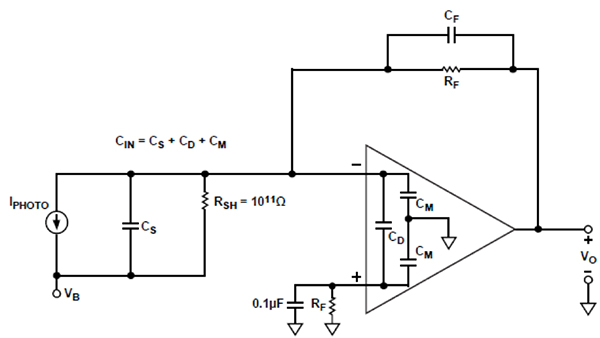Figure 2: Photodiode preamp equivalent circuit for AC and noise analysis (Image source: Analog Devices)

The design guidelines for this circuit are a full-scale output of 5 volts, with a maximum photodiode current of 200 μA. The full-scale output voltage and maximum photodiode current determine the value of the feedback resistor, per Equation 2: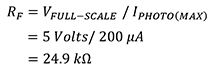Equation 2

There are three circuit design variables to consider in the stability analysis: the photodiode, the amplifier, and the parallel RF and CF (RF||CF) amplifier feedback network. The selection of the photodiode is due to its light response characteristics. However, its parasitic capacitance (CS) has a significant impact on the circuit’s noise gain and stability.

The network in Figure 2 has a direct influence on the stability as well as noise performance of the circuit. The op amp should have a low input bias current in the picoampere (pA) range, as with CMOS or FET input differential pairs. These differential transistor pairs preserve low input picoampere bias currents, and tens to hundreds of microvolts offset errors. If either or both of these errors are large, a non-linear behavior creeps into the amplifier’s response to the LED/photodiode results.

Additionally, the amplifier’s input common mode (CM) and differential mode (CD) parasitic capacitors can adversely affect the stability and overall accuracy of the system.

A reasonably stable bandwidth relies on RF, the gain-bandwidth product of the amplifier, and the total capacitance at the amplifier’s summing junction, CIN. The total amplifier summing junction capacitance, which includes the photodiode (SFH 2701) parasitic capacitance and the actual amplifier (Analog Devices’ AD8065ARTZ-R2) input capacitance (both differential and common mode), is calculated using Equation 3: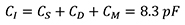Equation 3

Where:

CIN = total summing junction capacitance

CS = photodiode parasitic capacitance = 1.7 pF

CD = amplifier input differential capacitance = 4.5 pF

CM = amplifier input common-mode capacitance = 2.1 pF

In this article, the value of CS is the photodiode parasitic capacitance that results from a 5 volt reverse bias.

The amplifier’s gain-bandwidth product is 65 megahertz (MHz) (fCR). The AD8065’s maximum attainable bandwidth is larger than the design goal’s bandwidth of 2 MHz, which makes the AD8065 is a good candidate for a pulse oximeter circuit.

To validate the acceptable AD8065 bandwidth, Equation 4 defines the signal bandwidth that has a 45° phase margin (f (45)):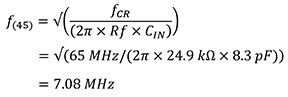Equation 4

Where:

f (45) = system signal bandwidth with a 45° phase margin

fCR = the amplifier’s gain bandwidth product

The value of f(45) exceeds the design bandwidth of 2 MHz.

The RF and CIN pole in the loop transfer function of the amplifier can produce peaking and instability. The addition of CF creates a zero in the loop transfer function which compensates for the pole’s effect and reduces the signal bandwidth (Figure 3).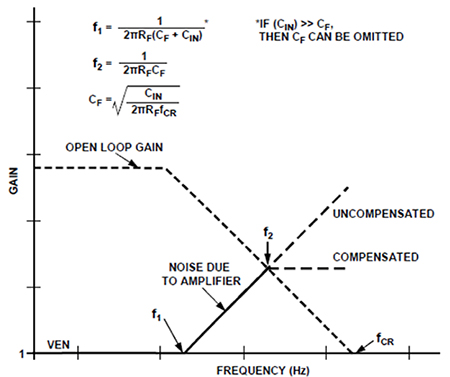Figure 3: Frequency response of the photodiode amplifier circuit using the parasitic input capacitance, CIN. (Image source: Analog Devices)

Equation 5 uses the corner frequency at f2 (2 MHz) to define the value of Cf: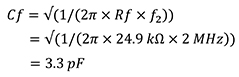Equation 5

To verify that 3.3 pF is enough capacitance to stabilize the system, Equation 6 calculates Cf for a 45° phase margin: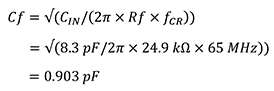Equation 6

The Cf = 3.3 pF value for the intended 2 MHz signal bandwidth is larger than the amplifier’s Cf = 0.903 pF. This lower value capacitance indicates that the system is stable because an increasing feedback capacitance increases the phase margin.

## Photodiode response time

The three factors that influence the photodiode response time are:

• Photodiode depleted region carrier charge collection time
• Photodiode undepleted region carrier charge collection time
• The photodiode/circuit combination resistor-capacitor (RC) time constant

The junction capacitance depends upon the diffused area of the photodiode and the applied reverse bias, so the rise times increase as the diffused area becomes smaller and as the reverse bias increases. The SFH 2701 PIN photodiode’s junction capacitance is 5 pF maximum for 0 volt bias. For a 1 volt reverse bias, the typical capacitance is 2 pF, and for 5 volts reverse bias it is 1.7 pF. For the purposes of this discussion, measurements were all taken with a reverse bias of 5 volts.

A characteristic of photodiodes is that under reverse bias conditions (photoconductive mode) with no illumination, a small amount of current called “dark current” flows through the photodiode. This needs to be compensated for using a second, identical photodiode (Figure 4). The second diode is shielded from the incoming LED lights and is connected to the noninverting input of the op amp to nullify the effect of the first diode’s dark current.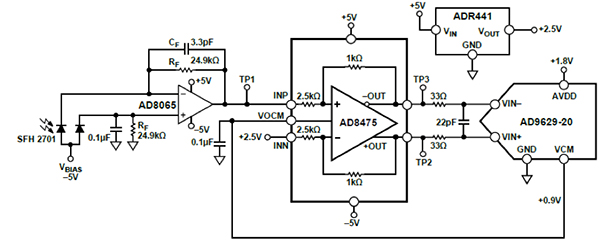Figure 4: Complete photo sensing circuit with dark current compensation diode in parallel with the input photodiode, SFH 2710. (Image source: Analog Devices)

This implementation of dark current compensation includes the parallel photodiode at the amplifier’s input and across SFH 2701, the additional Rf 24.9 kΩ resistor to match the RF feedback resistor, and the 0.1 mF capacitor to heavily reduce resistor noise.

The circuit takes the current from the high-speed SFH 2701 silicon PIN photodiode to drive the inputs of Analog Devices’ AD9629BCPZ-20 20 mega-sample-per-second (MSPS) analog-to-digital converter (ADC). This combination of devices provides:

• A bandwidth of 2 MHz
• A spectral sensitivity of 400 nm to 1050 nm
• A minimum of 49 nA of photocurrent sensitivity
• A dynamic range of 91 dB

The entire circuit draws 40 mA from the ±5 V supplies, making this configuration suitable for portable, battery-powered, high-speed, high-resolution, light intensity detection applications.

Pulse oximetry is one such application, but first circuit noise needs to be minimized.

## Pulse oximeter photo sensing input noise analysis

After selecting the components, the next task is to determine the overall system resolution. The noise contributors set the bottom floor of the resolution window. The noise sources combine in a root-sum-of-squares (RSS) manner.

In the photodiode preamp case, the dominant output noise sources are the op amp’s input voltage noise and the feedback resistor noise.

The calculation of the resistor noise uses the Johnson noise formula, Equation 7: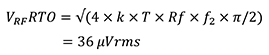Equation 7

Where:

k = Boltzmann’s constant (1.38 × 10-23J/K)

T = the absolute temperature in degrees Kelvin

p/2 approximates a single-pole bandwidth for f2

The primary output noise sources are the op amp’s input voltage noise and the system’s noise gain peaking that occurs between f1 and fCR, Equation 8 (Figure 3).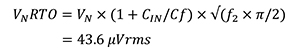Equation 8

Where VN = input voltage op amp noise (7 nV/√Hz).

The total rms noise referred to the output of AD8065 is the RSS value of VRFRTO and VNRTO, Equation 9.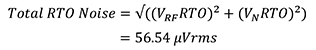Equation 9

The total output dynamic range of the preamp in decibels is the 20 times log10 of the full-scale output signal (5 volts) divided by the total output rms noise (56.54 μVRMS), as shown in Equation 10: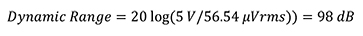Equation 10

## Selecting the ADC

The effective resolution is equal to the conversion of the maximum number of bits into the effective resolution. The maximum number of bits or the total number of codes is equal to the full-scale output divided by the total output noise, Equation 11: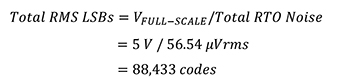Equation 11

The effective resolution is equal to the logarithm base 2 of the Total RMS LSBs, Equation 12: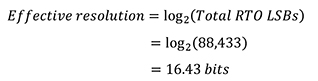Equation 12

The noise-free code resolution is equal to the effective resolution minus 2.7 bits, Equation 13: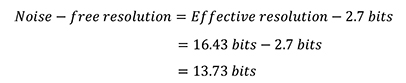Equation 13

Depending on the portable pulse oximeter’s specifications, 13 bits may meet or exceed the manufacturer’s requirements.

The dark current can be canceled using a second photodiode if the system’s LSB size is smaller than the contribution of dark current. For example, in a 16-bit resolution environment, the photocurrent LSB equals the maximum photocurrent divided by 2 to the number of bits, Equation 14.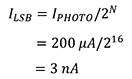Equation 14

The specification for the maximum amount of SFH 2701 dark current is 5 nA @ 25°C. Consequently, a 16-bit design needs compensation. This pulse oximeter application uses a 12-bit ADC; therefore, the LSB size is 49 nA, and so it does not require dark current compensation. Be aware that dark current increases ~10x every 20°C. So, a photodiode’s 5 pA dark current at 25°C becomes 50 pA at 45°C.

A reasonable guideline is to select an ADC with a sampling rate that is 10 times greater or more than the system’s bandwidth of 2 MHz. If the pulse oximeter’s bandwidth is 2 MHz, an ideal ADC must sample at a rate of 20 MSPS or higher, with 12 bits of resolution.

The AD9629-20 is a good fit as a 20 MSPS ADC with 12 bits of resolution. However, this converter requires differential inputs, so the 5 V p-p single-ended AD8065 signal must be attenuated to a 2 V p-p differential signal. The AD8475 differential funnel amplifier accomplishes with a single-ended-to-differential conversion. An added bonus from the AD8475 is the common-mode level shifting and precision attenuation.

The AD8475 supports a maximum 10 MHz output voltage of 2 V p-p. Additionally, the AD8475 has a 500 μV maximum output offset, a 10 nV/√Hz differential output noise, and −112 dB total harmonic distortion plus noise (THD + N).

The full-scale output of the AD8065 (5 Vp-p) and the analog input span of the AD9629-20 (2 Vp-p) determines the AD8475 gain, Equation 15: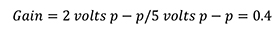Equation 15

An on-chip AD8475 common-mode voltage is 0.9 volts, which complements the AD9629-20’s VCM pin.

A final piece of the system’s noise puzzle is the noise contribution of the AD8475. The calculation of the AD8475 noise starts with first multiplying the AD8065 output noise by the AD8475 gain.

The AD8065 noise at AD8475’s output equals 0.4 × 43.6 μVRMS or 17 μVRMS. The AD8475 output noise is equal to the output noise density (10nV/√Hz) by the square root of the bandwidth (BW) of the output filter, Equation 16: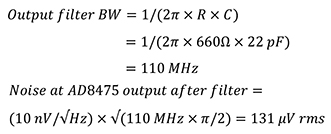Equation 16

Noise at AD8475 output after filter =

(10 nV/√Hz) x √ (110 MHz x p/2) = 131 mVRMS

The calculation of the total AD8475 output requires the RSS value of the AD8065 noise and AD8475 filtered output noise, per Equation 17: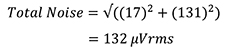Equation 17

The AD847 noise contribution enables the calculations of the system’s total RMS LSBs, effective resolution, Noise-Free Resolution, and the dynamic range, per Equation 18: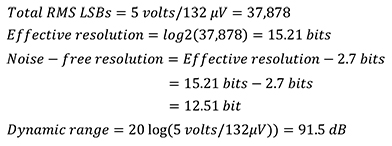Equation 18

## Test results

Theory is one thing, but the bench is where a designer really learns what is going on in the circuit.

For instance, a laser diode drives the D1 photodiode and generates a current. Photodiode D2 is in the circuit as a dark current compensation device and is covered with an optically opaque epoxy to prevent output current from D2 when D1 is excited. Forcing the photodiode to drive a larger than expected current, the approximate maximum rise and fall time of the AD8065 was 72 nanoseconds (ns) (Figure 5).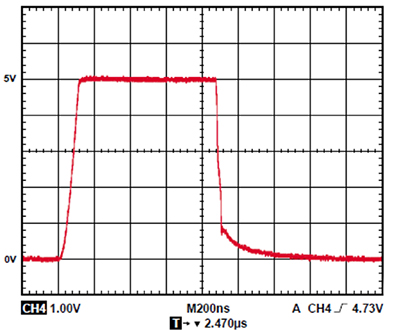Figure 5: Pulse response by overdriving the photodiode. (Image source: Analog Devices)

Figure 6 shows a screenshot of the CN0272 Evaluation Software successfully receiving conversion data from the AD9629-20 ADC and plotting the data in a chart.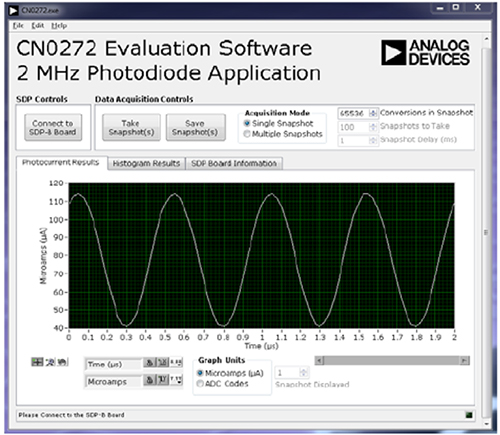Figure 6: Screenshot of CN0272 Evaluation Software Digitizing a 2 MHz varying light source. (Image source: Analog Devices)

Figure 7 shows the EVAL-CN0272-SDPZ evaluation board connected to the EVAL-SDP-CB1Z SDP board.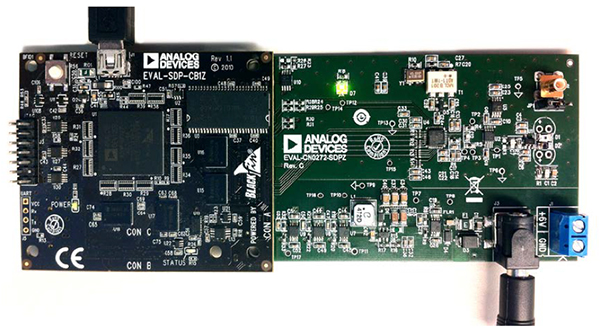Figure 7: EVAL-CN0272-SDPZ evaluation board connected to the EVAL-SDP-CB1Z SDP-B board. (Image source: Analog Devices)

## Conclusion

The wearable pulse oximeter measures blood oxygen saturation and pulse rate by sending LED signals through translucent parts of the body. The LED detection signal conditioning electronics require devices that complement optimum sensitivity, dynamic range, and bandwidth. The well understood traditional photodiode circuit addresses many key issues. However, the dynamic range is limited by the photodiode’s dark current.

In the proposed dark current compensation technique shown here, a second photodiode is added to the circuit to present a differential dark current signal which successfully subtracts out the error. Give it a try on your bench with Analog Devices’ EVAL-CN0272-SDPZ and EVAL-SDP-CB1Z SDP-B evaluation boards.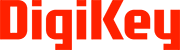Disclaimer: The opinions, beliefs, and viewpoints expressed by the various authors and/or forum participants on this website do not necessarily reflect the opinions, beliefs, and viewpoints of Digi-Key Electronics or official policies of Digi-Key Electronics.

### Achtergrondinformatie over deze auteurBonnie Baker

Bonnie Baker is een verdienstelijke auteur bij Digi-Key Electronics. Burr-Brown, Microchip en Texas Instruments zorgen er al meer dan 30 jaar voor dat ze intensief betrokken is bij analoge ontwerpen en systemen. Bonnie heeft een Masters of Science in Electrical Engineering van de Universiteit van Arizona (Tucson, AZ) en een bachelor in Muziek van de Universiteit van Noord-Arizona (Flagstaff, AZ). Naast haar fascinatie voor analoge ontwerpen heeft Bonnie ook een passie voor het delen van haar kennis en ervaring via het schrijven van al meer dan 450 artikelen, ontwerp- en applicatiebeschrijvingen.

### Over deze uitgever

De Noord-Amerikaanse redacteurs van Digi-Key